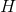# High School Physics : Enthalpy and Entropy

## Example Questions

### Example Question #1 : Enthalpy And Entropy

Enthalpy is a measure of __________.

total energy of a thermodynamic system

total work done by a thermodynamic system

the conversion of energy to heat

how easily something changes temperature

total energy lost in heat transfer

total energy of a thermodynamic system

Explanation:

Enthalpy, or, is the total energy of a thermodynamic system. Similar to how mechanical energy can change during mechanical processes, involving changing distances of velocities, enthalpy will increase or decrease with changes made to the thermodynamic state of the system. It is simply a measure for a different form of energy.

### Example Question #32 : Thermodynamics

An ice cube melts into water. While it is melting, what is the temperature of the mixture doing?

The temperature of the mixture steadily decreases while melting.

It remains constant while melting.

The temperature of the mixture steadily increases while melting.

The temperature fluctuates depending upon the point at which you measure it.

The ice part of the mixture is colder than the water part.

It remains constant while melting.

Explanation:

When an object is changing forms (solid to liquid in this case), the temperature remains constant. All of the energy that would normally go towards changing the internal temperature of the object is going into  the latent heat of fusion or enthalpy of fusion instead.

### Example Question #1 : Understanding Entropy

Entropy is a measure of __________.

temperature

energy within the system

disorder within the system

change in heat

specific heat